Test: Network Theorems - 2

# Test: Network Theorems - 2

Test Description

## 10 Questions MCQ Test Network Theory (Electric Circuits) | Test: Network Theorems - 2

Test: Network Theorems - 2 for Electrical Engineering (EE) 2022 is part of Network Theory (Electric Circuits) preparation. The Test: Network Theorems - 2 questions and answers have been prepared according to the Electrical Engineering (EE) exam syllabus.The Test: Network Theorems - 2 MCQs are made for Electrical Engineering (EE) 2022 Exam. Find important definitions, questions, notes, meanings, examples, exercises, MCQs and online tests for Test: Network Theorems - 2 below.
Solutions of Test: Network Theorems - 2 questions in English are available as part of our Network Theory (Electric Circuits) for Electrical Engineering (EE) & Test: Network Theorems - 2 solutions in Hindi for Network Theory (Electric Circuits) course. Download more important topics, notes, lectures and mock test series for Electrical Engineering (EE) Exam by signing up for free. Attempt Test: Network Theorems - 2 | 10 questions in 30 minutes | Mock test for Electrical Engineering (EE) preparation | Free important questions MCQ to study Network Theory (Electric Circuits) for Electrical Engineering (EE) Exam | Download free PDF with solutions
 1 Crore+ students have signed up on EduRev. Have you?
Test: Network Theorems - 2 - Question 1

### i1 = ?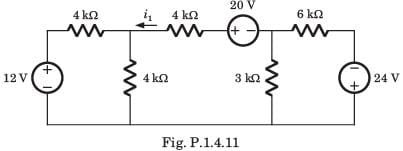Detailed Solution for Test: Network Theorems - 2 - Question 1

If we solve this circuit direct, we have to deal with three variable. But by simple manipulation variable can be reduced to one. By changing the LHS and RHS in Thevenin equivalent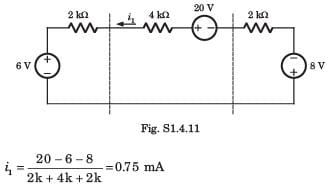Test: Network Theorems - 2 - Question 2

### A circuit is given in fig. P.1.4.12–13. Find the Thevenin equivalent as given in question..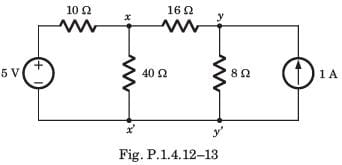Q. As viewed from terminal x and x' is

Detailed Solution for Test: Network Theorems - 2 - Question 2

We Thevenized the left side of xx' and source transformed right side of yy'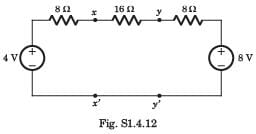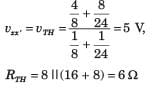Test: Network Theorems - 2 - Question 3

### A circuit is given in fig. P.1.4.12–13. Find the Thevenin equivalent as given in question..As viewed from terminal y and y' is

Detailed Solution for Test: Network Theorems - 2 - Question 3

Thevenin equivalent seen from terminal yy' is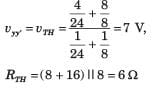Test: Network Theorems - 2 - Question 4

A practical DC current source provide 20 kW to a 50 Ω load and 20 kW to a 200 Ω load. The maximum power, that can drawn from it, is

Detailed Solution for Test: Network Theorems - 2 - Question 4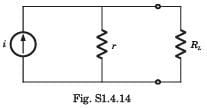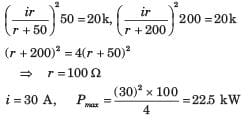Test: Network Theorems - 2 - Question 5

In the circuit of fig. P.1.4.15–16 when R = 0 Ω , the current iR equals 10 A.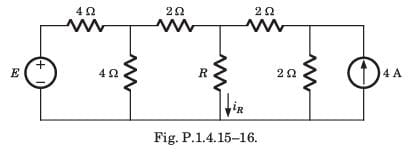Q. The value of R, for which it absorbs maximum power, is

Detailed Solution for Test: Network Theorems - 2 - Question 5

Thevenized the circuit across R, RTH = 2 Ω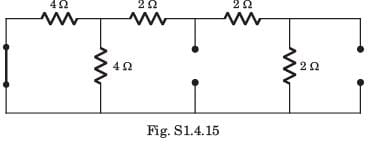Test: Network Theorems - 2 - Question 6

In the circuit of fig. P.1.4.15–16 when R = 0 Ω , the current iR equals 10 A.Q. The maximum power will be

Detailed Solution for Test: Network Theorems - 2 - Question 6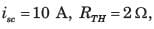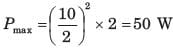Test: Network Theorems - 2 - Question 7

If vs1 = 6 V and vs 2 = -6 V then the value of vα is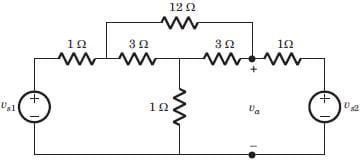Detailed Solution for Test: Network Theorems - 2 - Question 7

Since both source have opposite polarity, hence short circuit the all straight-through connection as shown in fig. S.1.4.33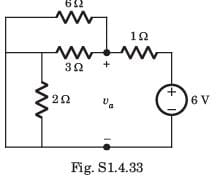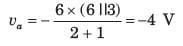Test: Network Theorems - 2 - Question 8

A network N feeds a resistance R as shown in fig.P1.4.34. Let the power consumed by R be P.If an identical network is added as shown in figure, the power consumed by R will be​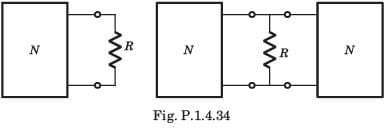Detailed Solution for Test: Network Theorems - 2 - Question 8

Let Thevenin equivalent of both network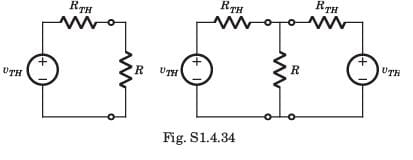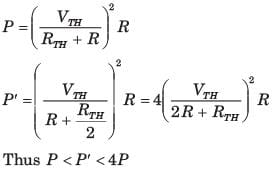Test: Network Theorems - 2 - Question 9

A certain network consists of a large number of ideal linear resistors, one of which is R and two constant ideal source. The power consumed by R is P1 when only the first source is active, and P2 when only the second source is active. If both sources are active simultaneously, then the power consumed by R is

Detailed Solution for Test: Network Theorems - 2 - Question 9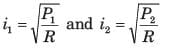using superposition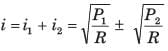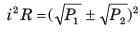Test: Network Theorems - 2 - Question 10

A battery has a short-circuit current of 30 A and an open circuit voltage of 24 V. If the battery is connected to an electric bulb of resistance 2 Ω, the power dissipated by the bulb is

Detailed Solution for Test: Network Theorems - 2 - Question 10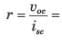0.8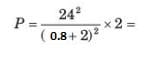146.93

## Network Theory (Electric Circuits)

23 videos|21 docs|27 tests
 Use Code STAYHOME200 and get INR 200 additional OFF Use Coupon Code
Information about Test: Network Theorems - 2 Page
In this test you can find the Exam questions for Test: Network Theorems - 2 solved & explained in the simplest way possible. Besides giving Questions and answers for Test: Network Theorems - 2, EduRev gives you an ample number of Online tests for practice

## Network Theory (Electric Circuits)

23 videos|21 docs|27 tests